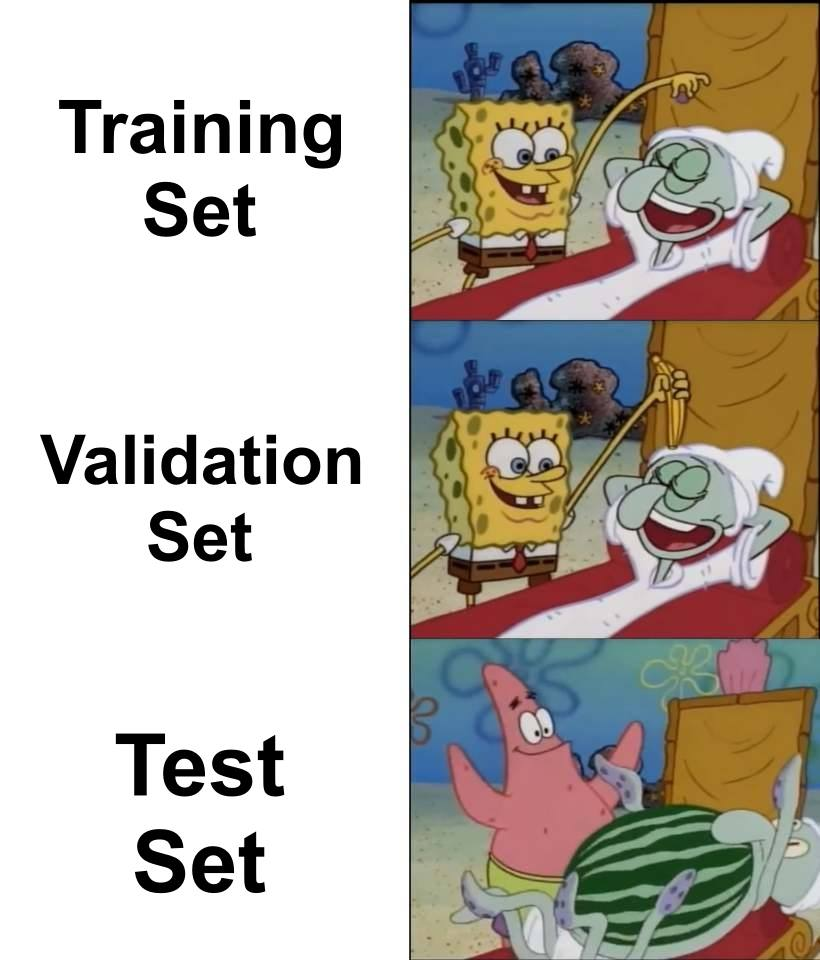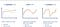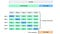# Overfitting : Identify and Resolve

It is better to be approximately right rather than precisely wrong.”- Warren BuffetSource: ML memes online

In Machine learning if the Model is focused on a particular training data so much that it missed the essential point then we consider the model is overfit. Hence it provides an answer which is far from correct. That means the accuracy is low. This model considers noise from the unrelated data as the signals which will affect the accuracy of the model. Even though the model is trained well which resulted in low loss, it will not help us and hence these kinds of models perform poorly with new Data. Underfitting is when the model has not captured the logic of data. Hence an under fitted model will have low accuracy and high loss. This can be illustrated by the following diagram.Source: overfitting online images

How to identify that our model is overfit?

While building the model, the data is split into 3 categories : Train, Validation and Test. The training data is used to train the model. Validation is used to test the built model at each step and Test data is used in the end to evaluate the model. Usually the ratios 80:10:10 or 70:20:10 are commonly used.

In the process of building the model, validation data is used at each epoch to test the model built until then. As a result we get the values of loss and accuracy of the model, also the validation loss and validation accuracy at each epoch. After the model is built we will test the model with the test data and get the accuracy. If this accuracy and validation accuracy has more difference then we can diagnose that our model is overfit.

If the loss is high in both validation and test set then the model is underfitting.

How to prevent overfitting:

1. Cross-Validation:

This is a very good approach to prevent overfitting. Here we generate multiple train test splits and tune the model. K fold validation is a standard cross validation where we divide data into k subsets. We hold 1 subset for validation and train the algorithm on the other subsets. Cross validation allows you to tune your hyper parameters. The performance is the average of all the values. This approach can be computationally expensive, but does not waste too much data. This process can be represented in the following diagram.Source: scikit website

2. Train with more data:

Training the model with more relevant data will help to identify the signal better and avoid noises as signals. Data augmentation is a way to increase training data. This can be achieved by flipping, translation, rotation, scaling, changing brightness, etc.

3. Remove Features:

This reduces the complexity of the model and also the noises can be avoided to an extent which will make the model more efficient . To decrease the complexity, we can remove layers or reduce the number of neurons to make the network smaller.

4. Early stopping:

When the model is being trained iteratively, we can measure the performance at each iteration. We should stop training the model when the Validation loss starts increasing. This way we can stop over fitting.

The following graph represents when to stop training the model.

5. Regularization:

Regularization is used to decrease the complexity of the model. This is done by penalizing the loss function and this is done in 2 ways L1 and L2.They can be represented by the following mathematical equations.

L1 Penalizes to optimize sum of absolute values of weights. It generates a model which is simple and interpretable. This is robust to outliers.

L2 penalizes the sum of square values of weights. This model is able to learn complex data patterns. This is not robust to outliers.

The both regularization techniques help to overcome overfitting and can be used depending on the need.

6. Drop out:

This is a type of regularization method which is used to disable the units of neural networks randomly. It can be implemented on any hidden layers or input layer but not the output layer. This prevents the dependency on other neurons in turn makes the network to learn independent correlations. It decreases the density of network illustrated in the following image.

Conclusion:

Overfitting is a problem to be taken care of because it will not let us use the existing data effectively. Sometimes this also can be estimated before building the models. By looking at the data, the ways it is collected, the way of sampling, the wrong assumptions, misrepresentations may signal overfitting. To avoid this , inspect the data properly before proceeding to model. Sometimes overfitting cannot be detected in preprocessing in such cases it can be detected after building the model. We can use a few of the above techniques to overcome Overfitting.

Originally published at https://www.numpyninja.com on January 11, 2021.

## Nerd For Tech

From Confusion to Clarification

Written by

## Mahitha Singirikonda

Data Scientist and Machine learning Engineer

## More From Medium

Medium is an open platform where 170 million readers come to find insightful and dynamic thinking. Here, expert and undiscovered voices alike dive into the heart of any topic and bring new ideas to the surface. Learn more

Follow the writers, publications, and topics that matter to you, and you’ll see them on your homepage and in your inbox. Explore

If you have a story to tell, knowledge to share, or a perspective to offer — welcome home. It’s easy and free to post your thinking on any topic. Write on Medium